# Unit 4 COA.pptx

22 de Mar de 2023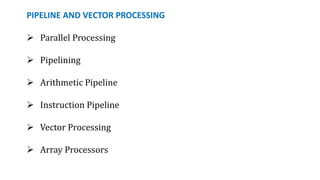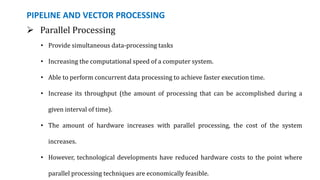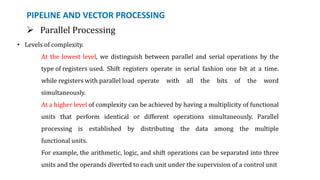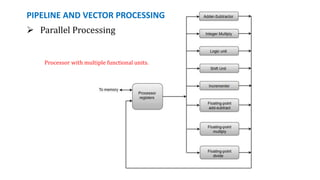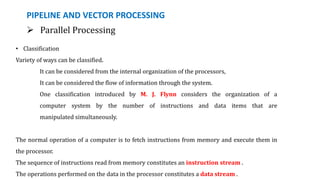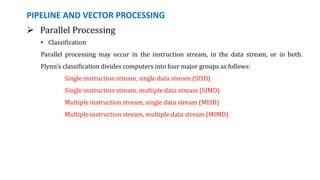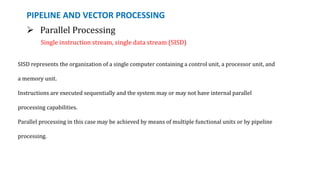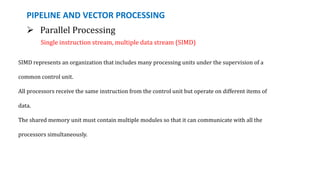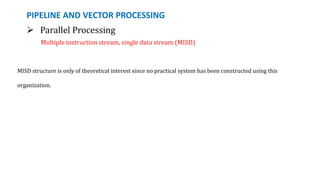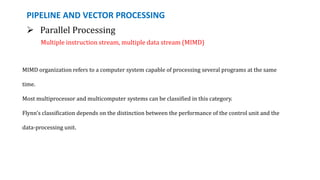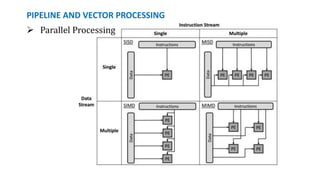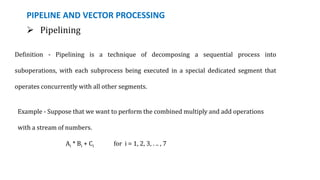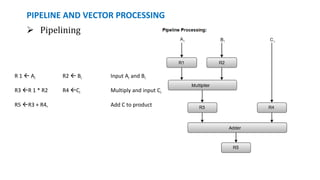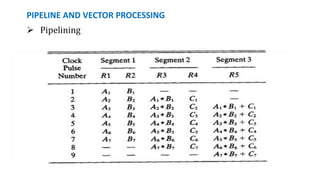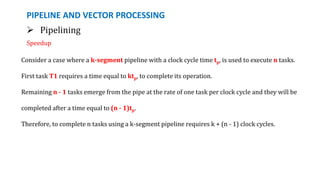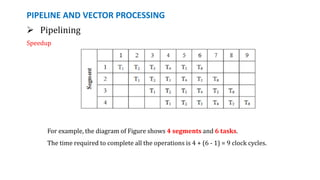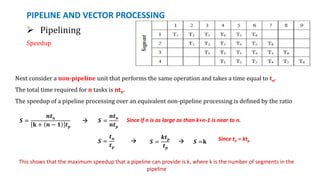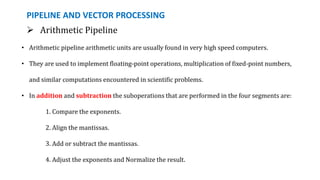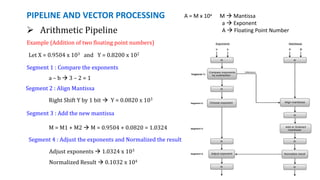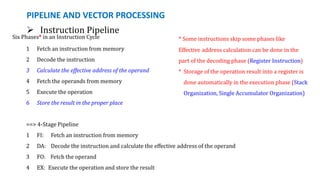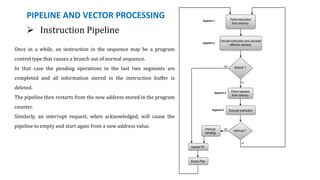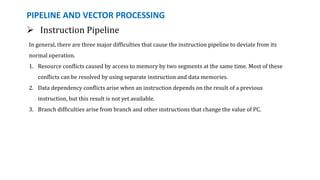1 de 23

### Unit 4 COA.pptx

1. PIPELINE AND VECTOR PROCESSING  Parallel Processing  Pipelining  Arithmetic Pipeline  Instruction Pipeline  Vector Processing  Array Processors
2. PIPELINE AND VECTOR PROCESSING  Parallel Processing • Provide simultaneous data-processing tasks • Increasing the computational speed of a computer system. • Able to perform concurrent data processing to achieve faster execution time. • Increase its throughput (the amount of processing that can be accomplished during a given interval of time). • The amount of hardware increases with parallel processing, the cost of the system increases. • However, technological developments have reduced hardware costs to the point where parallel processing techniques are economically feasible.
3. PIPELINE AND VECTOR PROCESSING  Parallel Processing • Levels of complexity. At the lowest level, we distinguish between parallel and serial operations by the type of registers used. Shift registers operate in serial fashion one bit at a time. while registers with parallel load operate with all the bits of the word simultaneously. At a higher level of complexity can be achieved by having a multiplicity of functional units that perform identical or different operations simultaneously. Parallel processing is established by distributing the data among the multiple functional units. For example, the arithmetic, logic, and shift operations can be separated into three units and the operands diverted to each unit under the supervision of a control unit
4. PIPELINE AND VECTOR PROCESSING  Parallel Processing Processor with multiple functional units.
5. PIPELINE AND VECTOR PROCESSING  Parallel Processing • Classification Variety of ways can be classified. It can be considered from the internal organization of the processors, It can be considered the flow of information through the system. One classification introduced by M. J. Flynn considers the organization of a computer system by the number of instructions and data items that are manipulated simultaneously. The normal operation of a computer is to fetch instructions from memory and execute them in the processor. The sequence of instructions read from memory constitutes an instruction stream . The operations performed on the data in the processor constitutes a data stream .
6. PIPELINE AND VECTOR PROCESSING  Parallel Processing • Classification Parallel processing may occur in the instruction stream, in the data stream, or in both. Flynn's classification divides computers into four major groups as follows: Single instruction stream, single data stream (SISD) Single instruction stream, multiple data stream (SIMD) Multiple instruction stream, single data stream (MISD) Multiple instruction stream, multiple data stream (MIMD)
7. PIPELINE AND VECTOR PROCESSING  Parallel Processing Single instruction stream, single data stream (SISD) SISD represents the organization of a single computer containing a control unit, a processor unit, and a memory unit. Instructions are executed sequentially and the system may or may not have internal parallel processing capabilities. Parallel processing in this case may be achieved by means of multiple functional units or by pipeline processing.
8. PIPELINE AND VECTOR PROCESSING  Parallel Processing Single instruction stream, multiple data stream (SIMD) SIMD represents an organization that includes many processing units under the supervision of a common control unit. All processors receive the same instruction from the control unit but operate on different items of data. The shared memory unit must contain multiple modules so that it can communicate with all the processors simultaneously.
9. PIPELINE AND VECTOR PROCESSING  Parallel Processing Multiple instruction stream, single data stream (MISD) MISD structure is only of theoretical interest since no practical system has been constructed using this organization.
10. PIPELINE AND VECTOR PROCESSING  Parallel Processing Multiple instruction stream, multiple data stream (MIMD) MIMD organization refers to a computer system capable of processing several programs at the same time. Most multiprocessor and multicomputer systems can be classified in this category. Flynn's classification depends on the distinction between the performance of the control unit and the data-processing unit.
11. PIPELINE AND VECTOR PROCESSING  Parallel Processing
12. PIPELINE AND VECTOR PROCESSING  Pipelining Definition - Pipelining is a technique of decomposing a sequential process into suboperations, with each subprocess being executed in a special dedicated segment that operates concurrently with all other segments. Example - Suppose that we want to perform the combined multiply and add operations with a stream of numbers. Ai * Bi + Ci for i = 1, 2, 3, . .. , 7
13. PIPELINE AND VECTOR PROCESSING  Pipelining R 1  Ai R2  Bi Input Ai and Bi R3 R 1 * R2 R4 Ci Multiply and input Ci R5 R3 + R4, Add C to product
14. PIPELINE AND VECTOR PROCESSING  Pipelining
15. PIPELINE AND VECTOR PROCESSING  Pipelining Speedup Consider a case where a k-segment pipeline with a clock cycle time tp, is used to execute n tasks. First task T1 requires a time equal to ktp, to complete its operation. Remaining n - 1 tasks emerge from the pipe at the rate of one task per clock cycle and they will be completed after a time equal to (n - 1)tp. Therefore, to complete n tasks using a k-segment pipeline requires k + (n - 1) clock cycles.
16. PIPELINE AND VECTOR PROCESSING  Pipelining Speedup For example, the diagram of Figure shows 4 segments and 6 tasks. The time required to complete all the operations is 4 + (6 - 1) = 9 clock cycles.
17. PIPELINE AND VECTOR PROCESSING  Pipelining Speedup 𝑺 = 𝒏𝒕𝒏 𝐤 + 𝒏 − 𝟏 𝒕𝒑  𝑺 = 𝒏𝒕𝒏 𝒏𝒕𝒑 Since if n is as large as than k+n-1 is near to n. Next consider a non-pipeline unit that performs the same operation and takes a time equal to tn. The total time required for n tasks is ntn. The speedup of a pipeline processing over an equivalent non-pipeline processing is defined by the ratio 𝑺 = 𝒕𝒏 𝒕𝒑  𝑺 = 𝒌𝒕𝒑 𝒕𝒑 𝑺 =k  Since tn = ktp This shows that the maximum speedup that a pipeline can provide is k, where k is the number of segments in the pipeline
18. PIPELINE AND VECTOR PROCESSING  Arithmetic Pipeline • Arithmetic pipeline arithmetic units are usually found in very high speed computers. • They are used to implement floating-point operations, multiplication of fixed-point numbers, and similar computations encountered in scientific problems. • In addition and subtraction the suboperations that are performed in the four segments are: 1. Compare the exponents. 2. Align the mantissas. 3. Add or subtract the mantissas. 4. Adjust the exponents and Normalize the result.
19. PIPELINE AND VECTOR PROCESSING  Arithmetic Pipeline Example (Addition of two floating point numbers) Let X = 0.9504 x 103 and Y = 0.8200 x 102 A = M x 10a M  Mantissa a  Exponent A  Floating Point Number Segment 1 : Compare the exponents a – b  3 – 2 = 1 Segment 2 : Align Mantissa Right Shift Y by 1 bit  Y = 0.0820 x 103 Segment 3 : Add the new mantissa M = M1 + M2  M = 0.9504 + 0.0820 = 1.0324 Segment 4 : Adjust the exponents and Normalized the result Adjust exponents  1.0324 x 103 Normalized Result  0.1032 x 104
20. PIPELINE AND VECTOR PROCESSING  Instruction Pipeline Six Phases* in an Instruction Cycle 1 Fetch an instruction from memory 2 Decode the instruction 3 Calculate the effective address of the operand 4 Fetch the operands from memory 5 Execute the operation 6 Store the result in the proper place ==> 4-Stage Pipeline 1 FI: Fetch an instruction from memory 2 DA: Decode the instruction and calculate the effective address of the operand 3 FO: Fetch the operand 4 EX: Execute the operation and store the result * Some instructions skip some phases like Effective address calculation can be done in the part of the decoding phase (Register Instruction) * Storage of the operation result into a register is done automatically in the execution phase (Stack Organization, Single Accumulator Organization)
21. PIPELINE AND VECTOR PROCESSING  Instruction Pipeline Once in a while, an instruction in the sequence may be a program control type that causes a branch out of normal sequence. In that case the pending operations in the last two segments are completed and all information stored in the instruction buffer is deleted. The pipeline then restarts from the new address stored in the program counter. Similarly, an interrupt request, when acknowledged, will cause the pipeline to empty and start again from a new address value.
22. PIPELINE AND VECTOR PROCESSING  Instruction Pipeline In the absence of a branch instruction, each segment operates on different instructions. Thus, in step 4, instruction 1 is being executed in segment EX; the operand for instruction 2 is being fetched in segment FO; instruction 3 is being decoded in segment DA; and instruction 4 is being fetched from memory in segment FL Assume now that instruction 3 is a branch instruction. As soon as this instruction is decoded in segment DA in step 4, the transfer from FI to DA of the other instructions is halted until the branch instruction is executed in step 6. If the branch is taken, a new instruction is fetched in step 7. If the branch is not taken, the instruction fetched previously in step 4 can be used. The pipeline then continues until a new branch instruction is encountered.
23. PIPELINE AND VECTOR PROCESSING In general, there are three major difficulties that cause the instruction pipeline to deviate from its normal operation. 1. Resource conflicts caused by access to memory by two segments at the same time. Most of these conflicts can be resolved by using separate instruction and data memories. 2. Data dependency conflicts arise when an instruction depends on the result of a previous instruction, but this result is not yet available. 3. Branch difficulties arise from branch and other instructions that change the value of PC.  Instruction Pipeline